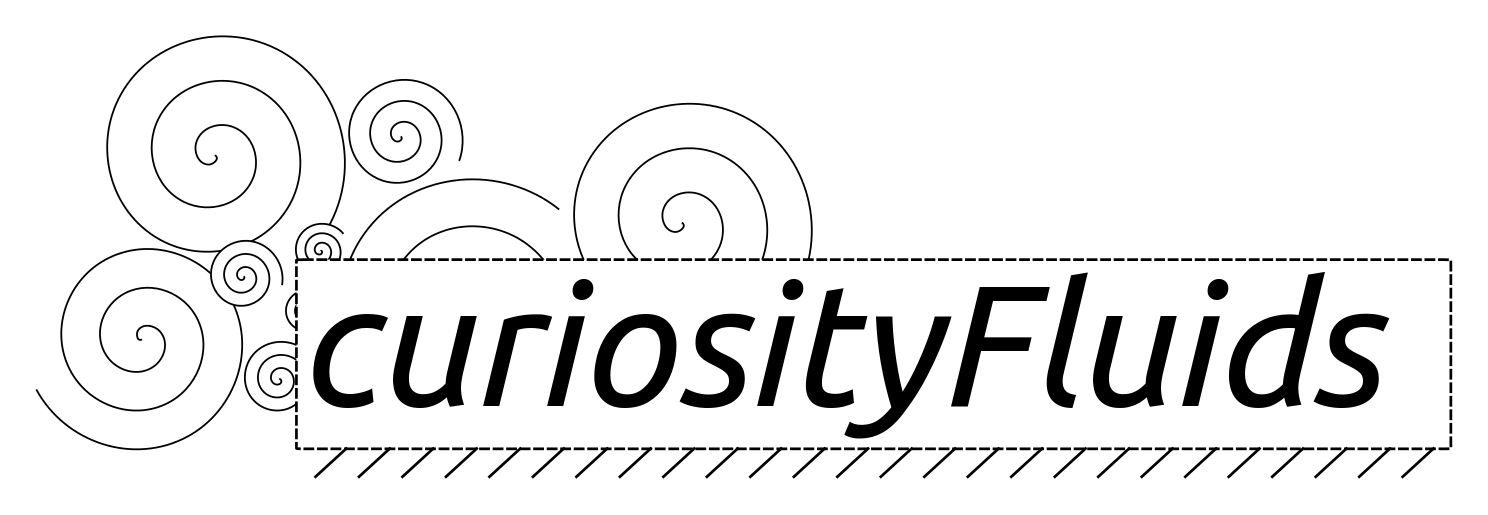Skip to content# Derivation of the Euler Equations

In this post I am going to derive the compressible Euler equations:$\frac{\partial \rho}{\partial t} + \nabla \cdot (\rho \vec{V}) =0$ (1)$\rho \frac{\partial \vec{V}}{\partial t} + \rho \vec{V} \cdot \nabla \vec{V} = -\nabla p$ (2)$\rho \dot{q} = \frac{\partial}{\partial t}\rho e + \nabla \cdot \left[\rho (h+\frac{|V|^2}{2})\right]$ (3)

These equations are essentially the conservation equations that we know from basic physics conservation of mass (1), conservation of momentum (2), and conservation of energy (3).

For a given system, these principles of conservation are summarized as:

1. Conservation of Mass:$\frac{dm}{dt}=0$
2. Conservation of linear momentum:$\vec{F}=m\vec{a}=\frac{d}{dt}m\vec{V}$
3. Conservation of energy:$\frac{dE}{dt}=\dot{Q}-\dot{W}$

The equations above refer to the net rate of change of conserved properties for the system. However it in fluid mechanics the analysis isn’t usually done on a system it is done on a control volume (See Figure). A control volume is a fixed volume within the system, surrounded by a control surface.In order to relate the conservation equations for the system to the fixed control volume we must use Reynolds Transport Theorem (I will do a separate post deriving Reynold’s transport theorem):

Reynolds transport theorem for some property X is:$\frac{D X}{Dt}=\frac{\partial}{\partial t} \iiint \limits_{C.V.} x \rho d\vee + \iint \limits_{C.S.} x(\rho\vec{V}\cdot d\vec{A})$

In the equation above, the lower case x refers to the amount of the conserved property per unit mass. So$X=xm$. Basically what Reynolds transport theorem says is that the total change of the property X is: The change in amount of X within the control volume with time the difference between the amount of X leaving and entering the control volume.

### Conservation of Mass

For conservation of mass, we know:$\frac{dm}{dt}=0$

The rate of change of mass for the control volume comes from Reynolds transport theorm. Here, the conserved variable X in Reynolds transport is obviously the mass m. Therefore the mass per unit mass is m/m and so x=1.

So Reynolds transport theorem gives:$\frac{Dm}{Dt} = \frac{\partial}{\partial t}\iiint \limits_{C.V.} \rho d\vee+ \iint \limits_{C.S.} \rho \vec{V} \cdot d\vec{A}$

Thus the final conservation of mass equation:$\frac{\partial}{\partial t}\iiint\limits_{C.V.} \rho d\vee+\iint\limits_{C.S.} \rho\vec{V}\cdot d\vec{A}=0$

### Conservation of Linear Momentum

For conservation of linear momentum we know:$\Sigma\vec{F}=m\vec{a}=\frac{d}{dt}m\vec{V}$

For Reynolds transport theorem the conserved property is the linear momentum, mV. Therefore the linear momentum per unit mass is just the velocity, V. This gives the equation for conservation of linear momentum:$\Sigma\vec{F} = \frac{\partial}{\partial t} \iiint \limits_{C.V.} \rho \vec{V} d\vee + \iint \limits_{C.S.} \vec{V}(\rho\vec{V}\cdot d\vec{A})$

Recall that$\Sigma F$ is the net force on the control volume. This can include body forces, pressure forces, and viscous forces. However, typically body forces are ignored. Additionally the Euler equations are used to analyze inviscid flows where shear forces are also ignored. Thus the force is just integral of the pressure around the control surface.$\frac{\partial}{\partial t} \iiint \limits_{C.V.} \rho \vec{V} d\vee + \iint \limits_{C.S.} \vec{V}(\rho\vec{V}\cdot d\vec{A}) = - \iint \limits_{C.S.} p d\vec{A}$

### Conservation of Energy

Conservation of energy gives:$\frac{dE}{dt}=\dot{Q}-\dot{W}$

In general, flows are assumed to contain only internal energy (u), and kinetic and potential energies. Therefore energy per unit mass, e=E/m, is given by:$e=E/m=u+\frac{|V|^2}{2}+gz$

Along with Reynolds transport, these equations combine to give:$\dot{Q}-\dot{W}=\frac{\partial}{\partial t} \iiint \limits_{C.V.} \rho e d\vee + \iint \limits_{C.S.} e(\rho\vec{V}\cdot d\vec{A})$

or$\dot{Q}-\dot{W}=\frac{\partial}{\partial t} \iiint \limits_{C.V.} \rho e d\vee + \iint \limits_{C.S.}(u+\frac{|V|^2}{2}+gz)(\rho\vec{V}\cdot d\vec{A})$

This is almost the final form of conservation of energy. However, it needs to be recognized that work is required to push the mass across the control volume boundaries. This is called flow work:

Flow work:$\iint\limits_{C.S.} \frac{p}{\rho}(\rho\vec{V}\cdot d\vec{A}$

Enthalpy (\$latex h=\frac{p}{\rho}) is the combination of internal energy and flow work. Therefore the final form of the energy equation is:$\dot{Q}-\dot{W}=\frac{\partial}{\partial t} \iiint \limits_{C.V.} \rho e d\vee + \iint \limits_{C.S.}(h+\frac{|V|^2}{2})(\rho\vec{V}\cdot d\vec{A})$

### Differential Form of the Equations

We have now derived the conservation of mass, linear momentum, and energy for a control volume (in integral form). It is useful however to represent these equations in differential form as PDEs. To do this we use divergence theorem.

Divergence theorem states that:$\iint\limits_A \vec{B} \cdot d \vec{A} = \iiint\limits_\vee \nabla \cdot \vec{B} d\vee$ (for a vector$\vec{B}$

and$\iint\limits_A \phi \cdot d \vec{A} = \iiint\limits_\vee \nabla \phi d\vee$ (for a scalar$\phi$

We can apply these identities to the integral relations we already derived. For example in conservation of mass,$\iint \limits_{C.S.} \rho \vec{V} \cdot d\vec{A}$ can become$\iiint\limits_{C.V.}\nabla \cdot (\rho \vec{V}) d \vee$. This gives:$\frac{\partial}{\partial t}\iiint\limits_{C.V.} \rho d\vee+\iiint\limits_{C.V.}\nabla \cdot (\rho \vec{V}) d \vee=0$

which then becomes the final differential form of the conservation of mass equation:$\frac{\partial \rho}{\partial t} + \nabla \cdot (\rho \vec{V}) =0$

Similarly for conservation of linear momentum:$\rho \frac{\partial \vec{V}}{\partial t} + \rho \vec{V} \cdot \nabla \vec{V} = -\nabla p$

And (assuming no external work) for conservation of energy:$\rho \dot{q} = \frac{\partial}{\partial t}\rho e + \nabla \cdot \left[\rho (h+\frac{|V|^2}{2})\right]$

### Conclusion

Hopefully someone will find this useful! As usual if you find any mistakes (and there probably are some so beware) let me know!Categories

Basic Gas Dynamics

Tags